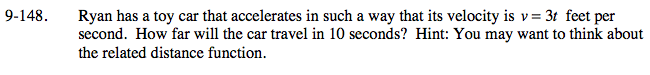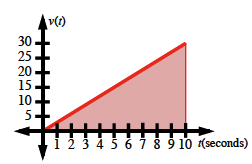### Home > PC > Chapter 9 > Lesson 9.3.4 > Problem9-148

9-148.

Ryan has a toy car that accelerates in such a way that its velocity is v = 3t feet per second. How far will the car travel in 10 seconds? Hint: You may want to think about the related distance function. Homework Help ✎Area under a velocity curve is distance.

$\text{Distance }=\left (\frac{1}{2} \right )(10)(30)=150 \text{ feet}$